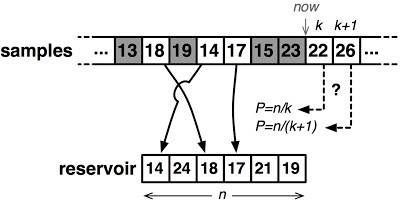# Select a random number from stream

Get FREE domain for 1st year and build your brand new siteIn this article, we describe an algorithm that is used to randomly select a number from a stream and show a prove that for a stream of n elements, all elements are chosen with the same final probability of 1/N.

1. Problem Statement
2. Reservoir sampling
3. Algorithm
4. Selecting random number from a stream
5. Naive Approach
6. Optimal Approach
7. Proof (sampling k elements)
8. Time and Space Complexity Analysis
9. Applications

Prerequisites:

## Problem Statement

Basic terms:

• A stream is a sequence of data.
• A randomized algorithm is an algorithm that makes random (or pseudorandom) choices.

The problem is to select a random number from a stream of N elements. The challenge is to keep the probability of 1/N and keep the space complexity of constant space O(1).

## Reservoir sampling

The idea is to create a 'reservoir' from a big ocean of data.

Definition: This is a randomized algorithm used to select k out of n samples where n is a very large unknown e,g search queries on a search engine.
This algorithm allows for sampling elements from a stream without knowing how many elements to expect.## Algorithm

1. Randomly select k items from a stream of items on unknown size.
2. Save the first k items in an array of size k.
3. For each item j, j > k
-> choose a random integer M from 1 to j(inclusive).
-> If M <- k, replace item M of the array with item j.

An algorithm is reservoir if it maintains the invariant that after each record is processed a true random sample size n can be extracted from the current state of the reservoir.

This algorithm exhibits a O(n) time complexity to select k elements with uniform probability.

## Selecting random number from a stream

Problem statement: Given a stream of numbers generate a random number from the stream.
In an optimal approach we see how to do this using constant space complexity by using the algorithm discussed above.
To rephrase we want to know how to generate a random number from the whole stream such that the probability of picking any number of 1/n using only O(1) space complexity.
That is to say at any point we could stop the stream and return k random elements.

## Naive Approach

We can process the stream and store all elements encountered in an array, and pick a random element from arr[0,...,arr.size() - 1].
This would make the space complexity O(N) for N items in the stream.

## Optimal Approach

We structure the reservoir using an array.
As we processes the stream we replace items in the reservoir using a probability.
To go about this, we first store the first element in the reservoir and iterate through the stream, for the ith element we make it the reservoir element with the probability of 1/i.

#### Algorithm:

1. Initialize count as 0, to store the count of numbers seen so far in a stream.
2. For each number x from the stream:
-> Increment count by 1
-> If count is 1, set result as x and return result
-> Generate a random number i from o to count - 1
-> If i is equal to count - 1, update the result as x.
``````#include<iostream>

using std::cout;
using std::endl;

int random(int x){
//random number generated
static int randN;
//keeps count of visted number of the stream
static int count = 0;
count++;

// if its is the first element in the stream
if(count == 1){
// we return it as the random number
randN = x;
}else{
// generate random number from 0 - count -1
int r = rand() % count;
// replace previous random number with new number with 1/count probability
if(r == count - 1)
randN = x;
}
//return random number
return randN;
}

int main(){
int stream[] = {3, 11, 23, 1, 7, 4, 8, 12, 6, 5, 9};
int n = sizeof(stream) / sizeof(stream);

//generate new seed
srand(time(NULL));

for(int i = 0; i < n; i++)
cout << i + 1 << " " << random(stream[i]) << endl;
return 0;
}
``````

## Proof (sampling k elements)

Lemma: For a stream of n elements, all elements are chosen with the same final probability of 1/n.

Base case: n = 1
The algorithm trivially works for n = 1.

Inductive Step: $\mathrm{n}\to \mathrm{n}+1$.
We want to show that for a stream of n + 1 elements, all items still have the same probability of $\frac{1}{\left(\mathrm{n}+1\right)}$ to be sampled.
In the algorithm we choose the next element of the stream with a probability of $\frac{1}{\left(\mathrm{n}+1\right)}$.
All other elements can be the current reservoir element with a probability of $\frac{1}{\mathrm{n}}$ by the inductive assumption.

The current reservoir element has a probability of 1 - $\frac{1}{\left(\mathrm{n}+1\right)}$ = $\frac{n}{\left(\mathrm{n}+1\right)}$ for staying in the reservoir.
Therefore all previous elements have a final probability of $\frac{1}{\mathrm{n}}$ * $\frac{n}{\left(\mathrm{n}+1\right)}$ = $\frac{1}{\left(\mathrm{n}+1\right)}$ to be the reservoir element after this step.
Therefore we conclude that all elements will have the same probability of being selected as the reservoir element.
full proof

## Time and Space Complexity Analysis

This computation takes O(n) time complexity for a stream of size n.

The space complexity is constant.

## Applications

• Database query planning.
• Sampling from streams e,g search queries from a search engine to determine weights of a search.

With this article at OpenGenus, you must have the complete idea of how to Select a random number from stream.